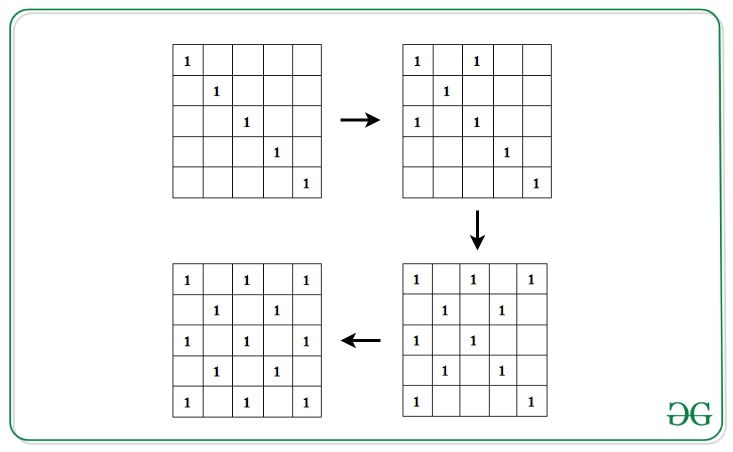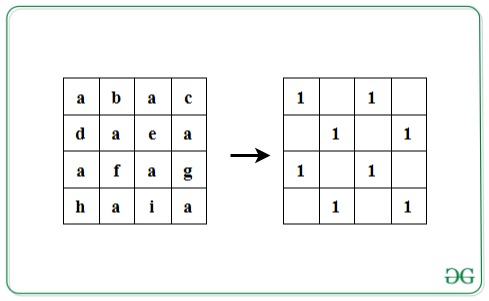# Check if given string satisfies the following conditions

• Last Updated : 23 Apr, 2021

Given a string S of length L, where L is a perfect square, the task is to check if the given string satisfies the following conditions:

• Insert the characters of the string into a square matrix A[][] of dimensions √L x √L in row-wise manner.
• Initialize another matrix M[][] with 0s. Fill the left diagonal with 1. Now, for 1 present in the left diagonal, fill its corresponding right diagonal with 1s.Attention reader! Don’t stop learning now. Get hold of all the important DSA concepts with the DSA Self Paced Course at a student-friendly price and become industry ready.  To complete your preparation from learning a language to DS Algo and many more,  please refer Complete Interview Preparation Course.

In case you wish to attend live classes with experts, please refer DSA Live Classes for Working Professionals and Competitive Programming Live for Students.

• Now, check if all the indices in matrix M[][] which contains 1, contains the same character in A[][].

If the condition is satisfied, print “Yes”. Otherwise, print “No”.

Examples:

Input: S = ”abacdaeaafaghaia”
Output: Yes
Explanation:Input: S = ”abacdaeabfaghaia”
Output: No

Approach: The idea is to traverse the matrix A[][] where its corresponding character in the matrix M[][] is 1. Follow the steps below to solve the problem:

• Calculate the dimensions of the matrix as N = √L.
• Iterate over the left diagonal by visiting each cell, A[i][i] where 1<= i<= N.
• For every element of the left diagonal at cell A[i][i], initialize variables x and y with i and traverse its corresponding right diagonal by visiting the character S[x*N + y] and S[y*N + x] and decrement x each time by 1 and increment y each time by 1 to move along the next cells in the right diagonals, while x is not smaller than 0 and y is smaller than N.
• If all characters are found to be same in the above step, print “Yes”. Otherwise, print “No” if any mismatch is found.

Below is the implementation of the above approach:

## C++

 `// C++ program for the above approach` `#include ``using` `namespace` `std;` `// Function to check if given string``// satisfies the given conditions``void` `isValid(string s)``{` `    ``// Dimensions``    ``int` `n = ``sqrt``(s.length());` `    ``char` `check = s;` `    ``// Left diagonal``    ``for` `(``int` `i = 0; i < n; i++) {``        ``int` `x = i, y = i;` `        ``// Right diagonal``        ``while` `(x >= 0 && y < n) {``            ``if` `(s[(n * x) + y] != check``                ``|| s[(n * y) + x] != check) {` `                ``// Conditions not satisfied``                ``cout << ``"No"` `<< endl;``                ``return``;``            ``}``            ``x--;``            ``y++;``        ``}``    ``}` `    ``// Print Yes``    ``cout << ``"Yes"` `<< endl;``}` `// Driver Code``int` `main()``{` `    ``// Given String``    ``string str = ``"abacdaeaafaghaia"``;` `    ``// Function call``    ``isValid(str);` `    ``return` `0;``}`

## Java

 `// Java program for the above approach``import` `java.util.*;``  ` `class` `GFG{``  ` `// Function to check if given string``// satisfies the given conditions``static` `void` `isValid(String s)``{``    ` `    ``// Dimensions``    ``int` `n = (``int``)Math.sqrt(s.length());``     ` `    ``char` `check = s.charAt(``0``);``     ` `    ``// Left diagonal``    ``for``(``int` `i = ``0``; i < n; i++)``    ``{``        ``int` `x = i, y = i;``        ` `        ``// Right diagonal``        ``while` `(x >= ``0` `&& y < n)``        ``{``            ``if` `(s.charAt((n * x) + y) != check ||``                ``s.charAt((n * y) + x) != check)``            ``{``                ` `                ``// Conditions not satisfied``                ``System.out.print(``"No"``);``                ``return``;``            ``}``            ``x--;``            ``y++;``        ``}``    ``}``    ` `    ``// Print Yes``    ``System.out.print(``"Yes"``);``}``  ` `// Driver Code``public` `static` `void` `main(String[] args)``{``    ` `    ``// Given String``    ``String str = ``"abacdaeaafaghaia"``;``    ` `    ``// Function call``    ``isValid(str);``}``}` `// This code is contributed by code_hunt`

## Python3

 `# Python3 program for the above approach``import` `math` `# Function to check if given string``# satisfies the given conditions``def` `isValid(s):``    ` `    ``# Dimensions``    ``n ``=` `int``(math.sqrt(``len``(s)))``    ``check ``=` `s[``0``]` `    ``# Left diagonal``    ``for` `i ``in` `range``(n):``        ``x ``=` `i``        ``y ``=` `i``        ` `        ``# Right diagonal``        ``while` `(x >``=` `0` `and` `y < n):``            ``if` `(s[n ``*` `x ``+` `y] !``=` `check ``or``                ``s[n ``*` `x ``+` `x] !``=` `check):``                    ` `                ``# Conditions not satisfied``                ``print``(``"No"``)``                ``return``                ` `            ``x ``-``=` `1``            ``y ``+``=` `1``            ` `    ``# Print Yes``    ``print``(``"Yes"``)``    ` `# Driver Code` `# Given String``str` `=` `"abacdaeaafaghaia"` `# Function call``isValid(``str``)` `# This code is contributed by avanitrachhadiya2155`

## C#

 `// C# program for the above approach ``using` `System;`` ` `class` `GFG{`` ` `// Function to check if given string``// satisfies the given conditions``static` `void` `isValid(``string` `s)``{``    ` `    ``// Dimensions``    ``int` `n = (``int``)Math.Sqrt(s.Length);``    ` `    ``char` `check = s;``    ` `    ``// Left diagonal``    ``for``(``int` `i = 0; i < n; i++)``    ``{``        ``int` `x = i, y = i;``        ` `        ``// Right diagonal``        ``while` `(x >= 0 && y < n)``        ``{``            ``if` `(s[(n * x) + y] != check ||``                ``s[(n * y) + x] != check)``            ``{``                ` `                ``// Conditions not satisfied``                ``Console.Write(``"No"``);``                ``return``;``            ``}``            ``x--;``            ``y++;``        ``}``    ``}`` ` `    ``// Print Yes``    ``Console.Write(``"Yes"``);``}` `// Driver code``public` `static` `void` `Main()``{``    ` `    ``// Given String``    ``string` `str = ``"abacdaeaafaghaia"``;`` ` `    ``// Function call``    ``isValid(str);``}``}` `// This code is contributed by sanjoy_62`

## Javascript

 ``
Output:
`Yes`

Time Complexity: O(L) where L is the length of the given string.
Auxiliary Space: O(L)

My Personal Notes arrow_drop_up